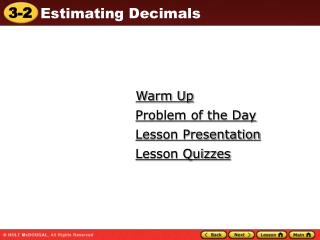DownloadDownload PresentationWarm Up

# Warm Up

Download Presentation## Warm Up

- - - - - - - - - - - - - - - - - - - - - - - - - - - E N D - - - - - - - - - - - - - - - - - - - - - - - - - - -
##### Presentation Transcript

1. Warm Up Problem of the Day Lesson Presentation Lesson Quizzes

2. Warm Up Order the decimals from least to greatest. 1. 18.74, 18.7, 18.47 2. 9.06, 9.66, 9.6, 9.076 Write each in words. 3. 3.072 4. 6.1258 18.47, 18.7, 18.74 9.06, 9.076, 9.6, 9.66 three and seventy-two thousandths six and one thousand two hundred fifty-eight ten-thousandths

3. Problem of the Day Calculate your age in months. Possible answer: 11 yr 8 mo = 140 mo

4. Vocabulary clustering front-end estimation

5. When numbers are about the same value, you can use clustering to estimate. Clustering means rounding the numbers to the same value.

6. The addends cluster around 170. To estimate the total number of calories, round each addend to 170. Add. Additional Example 1: Health Application Nancy wants to cycle, ice skate, and water ski for 30 minutes each. About how many calories will she burn in all? (Cycling = 165.5 calories, ice skating = 177.5 calories, and water skiing = 171.5 calories) 165.5 170 177.5 170 171.5 + 170 510 Nancy burns about 510 calories.

7. Check It Out: Example 1 Abner wants to run, roller-skate, and snow ski for 60 minutes each. About how many calories will he burn in all? (Running = 185.5 calories, roller-skating = 189.5 calories, and snow skiing = 191.5 calories.) 190 × 3 = 570 calories

8. Caution! • Look at the digit to the right of the place to which you are rounding. • If it is 5 or greater, round up. • If it is less than 5, round down.

9. Round to the nearest whole number. The sum is about 12. Round to the tenths. Align. – 0.2683 –0.3 Subtract. Additional Example 2: Rounding Decimals to Estimate Sums and Differences Estimate by rounding to the indicated place value. A. 7.13 + 4.68; ones B. 9.705 – 0.2683; tenths 7.13 + 4.68 7 + 5 = 12 9.705 9.7 9.4

10. Check It Out: Example 2 Estimate by rounding to the indicated place value. 6.09 + 3.72; ones 6 + 4 = 10

11. Remember! Compatible numbers are close to the numbers that are in the problem and are helpful when you are solving the problem mentally.

12. 35 and 2 are compatible. 75 and 25 are compatible. Additional Example 3: Using Compatible Numbers to Estimate Products and Quotients Estimate each product or quotient. A. 33.83  1.98 B. 72.77 ÷ 26.14 352 = 70 So 33.83  1.98 is about 70. 75 ÷ 25 = 3 So, 72.77 ÷ 26.14 is about 3.

13. Check It Out: Example 3 Estimate each product or quotient. 62.31 ÷ 18.52 60 ÷ 20 = 3

14. You can also use front-end estimation to estimate with decimals. Front-end estimation means to use only the whole-number part of the decimal.

15. Add the whole numbers only. The whole-number values of the decimals are less than the actual numbers, so the answer is an underestimate. Additional Example 4: Using Front-End Estimation Estimate a range for the sum. 7.86 + 36.97 + 5.40 Use front-end estimation. 7.86 7 36.97 36 5.40 + 5 at least 48 The exact answer of 7.86 + 36.97 + 5.40 is greater than 48.

16. Add the adjusted decimal part of the numbers. Add the whole-number estimate and this sum. The adjusted decimals are greater than the actual decimal, so 50.50 is an overestimate. Additional Example 4 Continued You can estimate a range for the sum by adjusting the decimal part of the numbers. Round the decimals up to 0.5 or 1. 0.86 1.00 0.97 1.00 + 0.50 0.40 2.50 48.00 + 2.50 = 50.50 The estimated range for the sum is from 48.00 to 50.50.

17. Check It Out: Example 4 Estimate a range for the sum. 8.92 + 47.88 + 3.41 underestimate: 8 + 47 + 3 = 58 overestimate: 9 + 48 + 3.5 = 60.5 from 58.00 to 60.50

18. Lesson Quizzes Standard Lesson Quiz Lesson Quiz for Student Response Systems

19. Lesson Quiz Estimate by rounding to the indicated place value. 1. 3.07442 + 1.352; tenths 2. 7.305 – 4.12689; nearest whole number 4.5 3 Estimate each product or quotient. 14 3. 6.75  1.82 3 4. 10.5 ÷ 3.42 5. The snowfall in December, January, and February was 18.26 cm, 29.36 cm, and 32.87 cm, respectively. About how many total centimeters of snow fell during the three months? 80 cm

20. Lesson Quiz for Student Response Systems 1. Estimate by rounding to the indicated place value. 5.891+9.326; hundredths A. 14.21 B. 14.22 C. 15.21 D. 15.22

21. Lesson Quiz for Student Response Systems 2. Estimate by rounding to the indicated place value. 29.35-17.92; nearest whole number A. 10 B. 11 C. 12 D. 13

22. Lesson Quiz for Student Response Systems 3. Identify the estimate of the product of 16.77  3.81. A. 48 B. 51 C. 64 D. 68

23. Lesson Quiz for Student Response Systems 4. Identify the estimate of the quotient of 17.43  3.91. A. 6 B. 5 C. 4 D. 3

24. Lesson Quiz for Student Response Systems 5. The weight of three students in a class are 48.23 pounds, 54.81 pounds, and 45.3 pounds. Identify the total weight of the students. A. 130 lb B. 140 lb C. 150 lb D. 160 lb